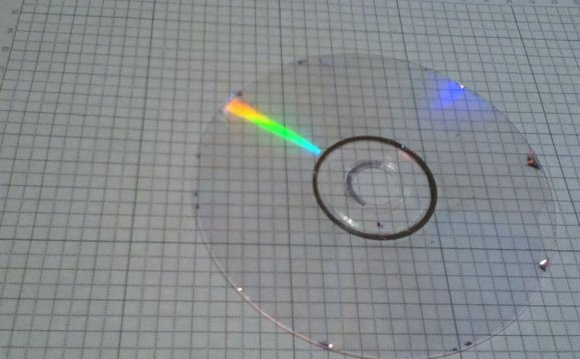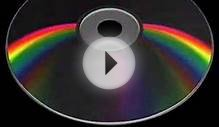# CD diffraction

May 18, 2022The information on a CD is stored on a very thin shiny spiral track. When light shines on these thin lines it is diffracted in all directions, the light from neighbouring lines interferes constructively when the sin of the angle=λ/d. This means that different colours of light are seen at different angles. This is what causes the coloured patterns that you might have seen. In this practical you will measure the angle of diffraction of different colours to determine the spacing of the lines on the CD.

The CD is acting as a reflecting diffraction grating. Each line is very thin and diffracts light at all angles as seen in the diagram on the right.

When brought to a focus in the eye parallel rays of light from each line interferes with light from the other lines. Constructive interference happens when the path difference is one whole wavelength so constructive interference will occur at different angles for each colour. Constructive interference happens when sinθ = λ/d where d is the line separation as shown below.

#### Method

The angle of the light source and the circular shape of the lines make the geometry of the diffraction patterns rather difficult to deal with however this is made much simpler if it is illuminated with light shining at 90°. If you then look directly at the centre you will see bright rings instead on the radial pattern seen above.

To view the rings stand with the light source behind you and hold the CD about 20cm from your eye then move it around so that the reflection of the bulb is in the centre. Now adjust the distance and angle so that you see clear coloured circles.

#### Measurement

Hold the CD on a ruler as shown then hold the ruler up to your eye and adjust as before so that you can see the rings. Move the CD backwards and forwards along the ruler so that the blue ring is on the edge of the CD, the radius of this ring is now the same as the radius of the CD (6cm). Now measure the distance from your eye to the CD on the ruler. The angle θ can be calculated by using trigonometry, tanθ=r/D.

Repeat with the other colours then using the values of wavelength for the colours given below use a graphical method to calculate the separation of the lines.

• Red 700 ± 80nm
• Orange 610 ± 10nm
• Yellow 580 ± 10nm
• Green 530 ± 50nm
• Blue 470 ± 20nm
• Violet 420 ± 30nm
Source: www.thinkib.net
##### RELATED VIDEOCDCD DiffractionCD Texture - Trying up the Diffraction